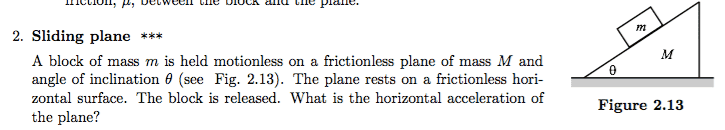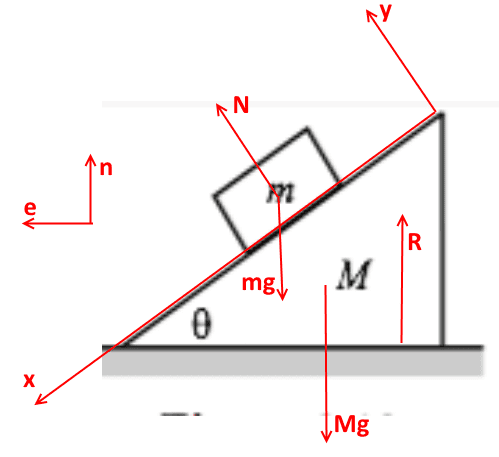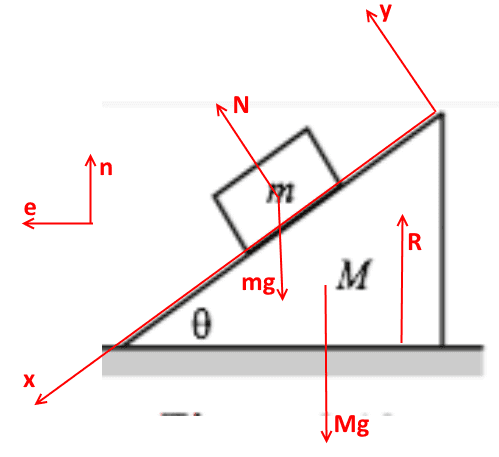# Normal contact force for a sliding slope

• I
• Happiness
In summary, the acceleration of ##M## depends on the net force and normal contact force between ##m## and ##M##, which is affected by the tightness of their surfaces. When ##M## is free to slide, the normal contact force ##N## may be smaller compared to when ##M## is fixed. The initial value of ##N## in both cases would be the same, but the equations of motion suggest that ##N## may be time dependent. The equations of motion also show that there are five unknowns in the system, and solving them using the summation of accelerations theorem can provide values for these unknowns. Alternatively, the problem can also be solved using Lagrange equations, and it can be extended

#### Happiness

For the following question, how do we know that the acceleration of ##M## is constant over time? And is the normal contact force between the two masses smaller as compared to that where ##M## is fixed?The acceleration depends on the net force on ##M##, which depends on the normal contact force ##N## between ##m## and ##M##. The force ##N## depends on how tightly the two surfaces are pressed together. So it seems plausible that ##N## is smaller when ##M## is free to slide compared to that when ##M## is fixed.

The initial velocities of ##m## and ##M## are zero. Suppose we set up the initial conditions by holding ##m## and ##M## fixed and then release them. Would the initial (when both masses are just released from grip) value of ##N## in this case be the same as the value of ##N## where ##M## is always fixed?

I guess they would be the same. But since the acceleration is constant over time, the value of ##N##, at all other time after ##M## starts to slide, must be the same as the initial value of ##N##. Then, we must conclude that the value of ##N## where ##M## is fixed is equal to the value of ##N## where ##M## is sliding away from ##m##. But when ##M## is sliding, it seems that the two masses are less tightly pressed together, and so ##N## should be smaller.

Last edited:
you should write the equations of motion to this system.

wrobel said:
you should write the equations of motion to this system.

I get ##N## is constant provided ##a_x## or ##A_x## or ##N'## is constant, where ##a## and ##A## are the accelerations of ##m## and ##M##, and ##N'## is the normal contact force on ##M## by the floor.

How do we know ##a_x## or ##A_x## or ##N'## is constant?

Suppose ##N## is velocity dependent. I believe the equations of motion, eg., ##N\cos\theta+Mg=N'##, still holds, just that now ##a_x##, ##A_x## and ##N'## will be time dependent. So it doesn't seem like we can deduce ##N## is time independent from the equations of motion.

Last edited:$$m\boldsymbol a_m=\boldsymbol N+m\boldsymbol g,\quad M\boldsymbol a_M=-\boldsymbol N+\boldsymbol R+M\boldsymbol g;\qquad (*)$$
$$\boldsymbol a_m=\boldsymbol a_M+w\boldsymbol e_x;\quad \boldsymbol N=N\boldsymbol e_y;$$
$$\boldsymbol n=\cos\theta\boldsymbol e_y-\sin\theta\boldsymbol e_x;\quad \boldsymbol e=\sin\theta \boldsymbol e_y+\cos\theta\boldsymbol e_x;$$
$$\boldsymbol a_M=a_M\boldsymbol e,\quad \boldsymbol R=R\boldsymbol n,\quad \boldsymbol g=-g\boldsymbol n$$
so we have four unknowns ##w,a_M,R,N## and four scalar equations (*)

wrobel said:$$m\boldsymbol a_m=\boldsymbol N+m\boldsymbol g,\quad M\boldsymbol a_M=-\boldsymbol N+\boldsymbol R+M\boldsymbol g;\qquad (*)$$
$$\boldsymbol a_m=\boldsymbol a_M+w\boldsymbol e_x;\quad \boldsymbol N=N\boldsymbol e_y;$$
$$\boldsymbol n=\cos\theta\boldsymbol e_y-\sin\theta\boldsymbol e_x;\quad \boldsymbol e=\sin\theta \boldsymbol e_y+\cos\theta\boldsymbol e_x;$$
$$\boldsymbol a_M=a_M\boldsymbol e,\quad \boldsymbol R=R\boldsymbol n,\quad \boldsymbol g=-g\boldsymbol n$$
so we have four unknowns ##w,a_M,R,N## and four scalar equations (*)

There should be 5 unknowns because ##m## could accelerate vertically as it slides down the slope.

Anyway, my question is how do we know the terms in the equations of motion are time independent?

Happiness said:
mm could accelerate vertically
sure, that has already been taken into account, look at the formulas carefully

wrobel said:
sure, that has already been taken into account, look at the formulas carefully

Shouldn't it be ##\mathbf {a_m}=\mathbf {a_M}+w\mathbf {e_x}+u\mathbf {e_y}##?

##M## is free to slide too. It accelerates to the right.

the term ##w\boldsymbol e_x## is the acceleration of ##m## relative to the wedge; ##\boldsymbol a_M## is acceleration of the wedge. We use the summation of accelerations theorem, the term ##u\boldsymbol e_y## is unnecessary

wrobel said:
the term ##w\boldsymbol e_x## is the acceleration of ##m## relative to the wedge; ##\boldsymbol a_M## is acceleration of the wedge. We use the summation of accelerations theorem, the term ##u\boldsymbol e_y## is unnecessary

What's the theorem about? The relative acceleration won't just be in the x direction, so I don't see why you only introduce a constant ##w##.

Anyway, this doesn't answer the question of time independence.

you do not understand banal things but what a self-confidence :)

wrobel said:
you do not understand banal things but what a self-confidence :)

You made a mistake isn't it? There should be 5 unknowns.

this continues posting #4. Solving linear system (*) we get
$$w=cg(m+M)\sin\theta,\quad a_M=-\frac{c}{2}mg\sin 2\theta,\quad R=cMg(M+m),\quad N=c mMg\cos\theta,$$
here $$c=\frac{1}{M+m\sin^2\theta}.$$ Calculations are in the attachment

Simpler way to solve this problem is to use the Lagrange equations. There is another problem of the same type but little bit more complicated : to replace the block with a disk that rolls on the wedge without slipping.

#### Attachments

• wedge and block.pdf
223.6 KB · Views: 298
Last edited: